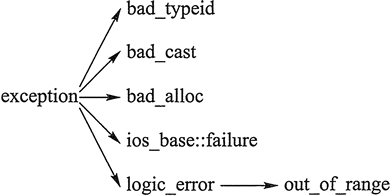## C++异常处理（try catch throw）完全攻略

• 内容
• 评论
• 相关

• 做除法的时候除数为 0；
• 用户输入年龄时输入了一个负数；
• 用 new 运算符动态分配空间时，空间不够导致无法分配；
• 访问数组元素时，下标越界；打开文件读取时，文件不存在。

## C++异常处理基本语法

C++ 通过 throw 语句和 try...catch 语句实现对异常的处理。throw 语句的语法如下：

throw  表达式;

try...catch 语句的语法如下：

try {
语句组
}
catch(异常类型) {
异常处理代码
}
...
catch(异常类型) {
异常处理代码
}

catch 可以有多个，但至少要有一个。

try...catch 语句的执行过程是：

• 执行 try 块中的语句，如果执行的过程中没有异常拋出，那么执行完后就执行最后一个 catch 块后面的语句，所有 catch 块中的语句都不会被执行；
• 如果 try 块执行的过程中拋出了异常，那么拋出异常后立即跳转到第一个“异常类型”和拋出的异常类型匹配的 catch 块中执行（称作异常被该 catch 块“捕获”），执行完后再跳转到最后一个 catch 块后面继续执行。

```#include <iostream>
using namespace std;
int main()
{
double m ,n;
cin >> m >> n;
try {
cout << "before dividing." << endl;
if( n == 0)
throw -1; //抛出int类型异常
else
cout << m / n << endl;
cout << "after dividing." << endl;
}
catch(double d) {
cout << "catch(double) " << d <<  endl;
}
catch(int e) {
cout << "catch(int) " << e << endl;
}
cout << "finished" << endl;
return 0;
}```

9 6↙
before dividing.
1.5
after dividing.
finished

9 0↙
before dividing.
catch\(int) -1
finished

## 能够捕获任何异常的 catch 语句

catch(...) {
...
}

```#include <iostream>
using namespace std;
int main()
{
double m, n;
cin >> m >> n;
try {
cout << "before dividing." << endl;
if (n == 0)
throw - 1;  //抛出整型异常
else if (m == 0)
throw - 1.0;  //拋出 double 型异常
else
cout << m / n << endl;
cout << "after dividing." << endl;
}
catch (double d) {
cout << "catch (double)" << d << endl;
}
catch (...) {
cout << "catch (...)" << endl;
}
cout << "finished" << endl;
return 0;
}```

9 0↙
before dividing.
catch (...)
finished

0 6↙
before dividing.
catch (double) -1
finished

## 异常的再拋出

```#include <iostream>
#include <string>
using namespace std;
class CException
{
public:
string msg;
CException(string s) : msg(s) {}
};
double Devide(double x, double y)
{
if (y == 0)
throw CException("devided by zero");
cout << "in Devide" << endl;
return x / y;
}
int CountTax(int salary)
{
try {
if (salary < 0)
throw - 1;
cout << "counting tax" << endl;
}
catch (int) {
cout << "salary < 0" << endl;
}
cout << "tax counted" << endl;
return salary * 0.15;
}
int main()
{
double f = 1.2;
try {
CountTax(-1);
f = Devide(3, 0);
cout << "end of try block" << endl;
}
catch (CException e) {
cout << e.msg << endl;
}
cout << "f = " << f << endl;
cout << "finished" << endl;
return 0;
}```

salary < 0
tax counted
devided by zero
f=1.2
finished

CountTa 函数拋出异常后自行处理，这个异常就不会继续被拋给调用者，即 main 函数。因此在 main 函数的 try 块中，CountTax 之后的语句还能正常执行，即会执行`f = Devide(3, 0);`

Devide 函数中拋出的异常被 main 函数中类型匹配的 catch 块捕获。第 38 行中的 e 对象是用复制构造函数初始化的。

```#include <iostream>
#include <string>
using namespace std;
int CountTax(int salary)
{
try {
if( salary < 0 )
throw string("zero salary");
cout << "counting tax" << endl;

}
catch (string s ) {
cout << "CountTax error : " << s << endl;
throw; //继续抛出捕获的异常
}
cout << "tax counted" << endl;
return salary * 0.15;
}
int main()
{
double f = 1.2;
try {
CountTax(-1);
cout << "end of try block" << endl;
}
catch(string s) {
cout << s << endl;
}
cout << "finished" << endl;
return 0;
}```

CountTax error:zero salary
zero salary
finished

## 函数的异常声明列表

void func() throw (int, double, A, B, C);

void func() throw (int, double, A, B, C){...}

void func() throw ();

## C++标准异常类

C++ 标准库中有一些类代表异常，这些类都是从 exception 类派生而来的。常用的几个异常类如图 1 所示。0条评论